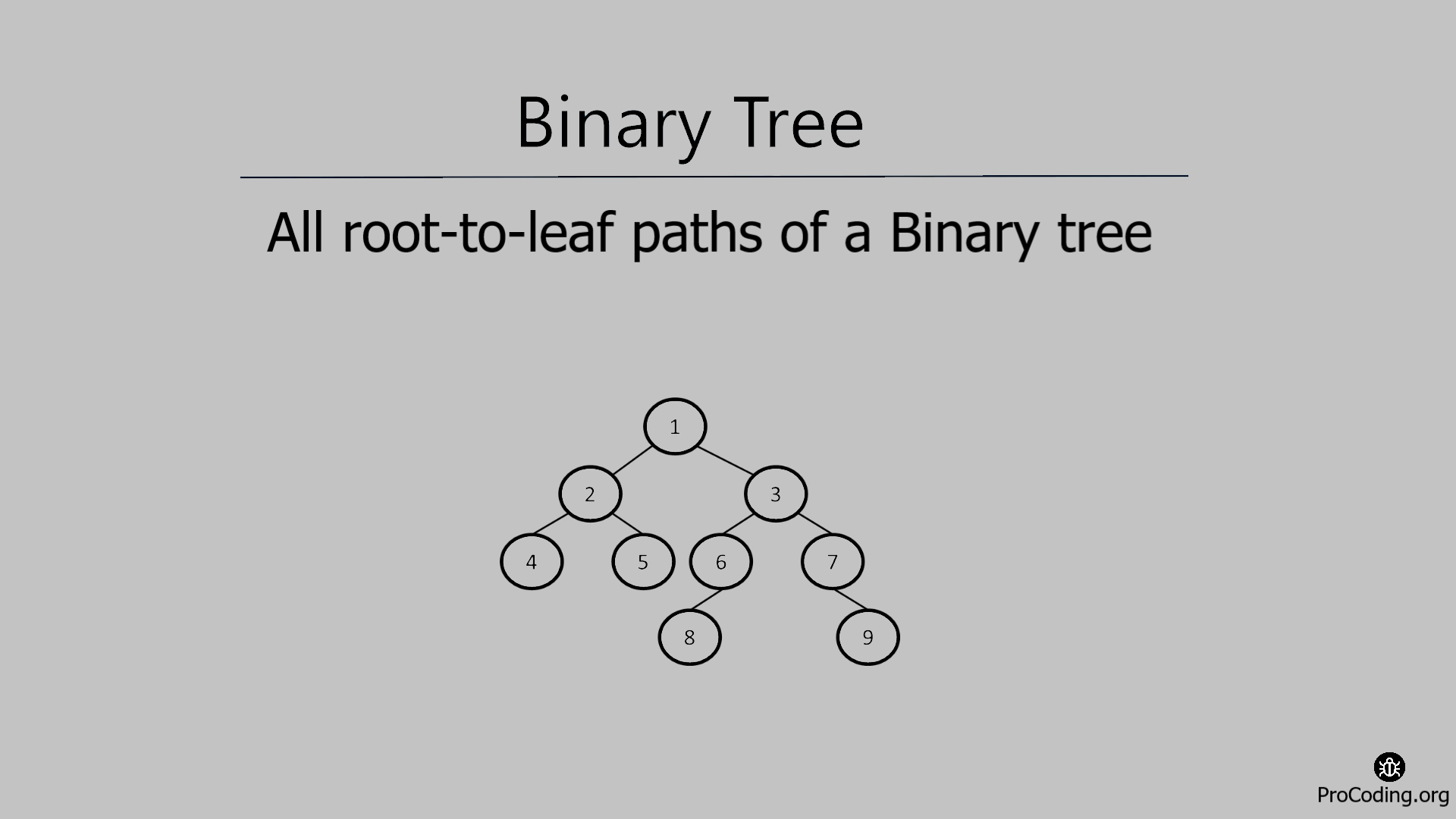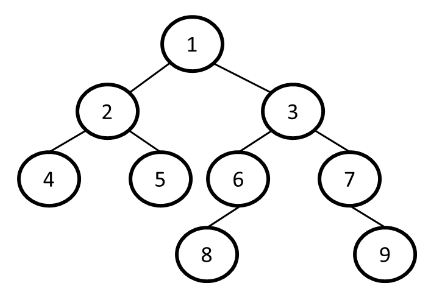# All root-to-leaf paths of a Binary tree

Abhishek Kumar Category: Data StructuresTags: datastructures tree binary-tree

Write a program to find all root to leaf paths of a Binary tree.
A tree has different paths from the root to each leaf node, print all the possible paths from the root to each leaf node separately.### All root-to-leaf paths of a Binary tree

A tree has different paths from the root to each leaf node. We have to print all the possible paths from the root to each leaf node separately.For this graph all possible root to leaf paths are

1. 1 -> 2 -> 4
2. 1 -> 2 -> 5
3. 1 -> 3 -> 6 -> 8
4. 1 -> 3 -> 7 -> 9
##### Example -
```Input:
1
/   \
2     3
/ \   / \
4   5 6   7

Output:
1 -> 2 -> 4
1 -> 2 -> 5
1 -> 3 -> 6
1 -> 3 -> 7
```

### Solution

For the solution, we have to traverse each node recursively and find all the possible paths for each leaf node from the root.

``````class Node:
def __init__(self, data):
self.left = None
self.data = data
self.right = None

def path_finder_util(root, string, paths):
if not root: return
string += str(root.data)
path_finder_util(root.left, string+'->', paths)
path_finder_util(root.right, string+'->', paths)
if not root.left and not root.right:
paths.append(string)

def path_finder(root):
if not root:
print("")
return
paths = []
path_finder_util(root, '', paths)
for path in paths:
print(path)

root = Node(1)
root.left = Node(2)
root.right = Node(3)
root.left.left = Node(4)
root.left.right = Node(5)
root.right.left = Node(6)
root.right.right = Node(7)
root.right.left.left = Node(8)
root.right.right.right = Node(9)
path_finder(root)``````
``````class Node {
constructor(data) {
this.left = null;
this.data = data;
this.right = null;
}
}

function pathFinderUtil(root, string, paths) {
if (root == null) return;
string = string + root.data;
pathFinderUtil(root.left, string + "->", paths);
pathFinderUtil(root.right, string + "->", paths);
if (root.left == null && root.right == null) {
paths.push(string);
}
}

function pathFinder(root) {
if (root == null) {
console.log("");
return;
}
let paths = [];
pathFinderUtil(root, "", paths);
for (let path of paths) {
console.log(path);
}
}

const root = new Node(1);
root.left = new Node(2);
root.right = new Node(3);
root.left.left = new Node(4);
root.left.right = new Node(5);
root.right.left = new Node(6);
root.right.right = new Node(7);
root.right.left.left = new Node(8);
root.right.right.right = new Node(9);
pathFinder(root);``````

#### Output

Output

```1->2->4
1->2->5
1->3->6->8
1->3->7->9```

In the above program, we have printed all the root-to-leaf paths as a string but we may need all paths as a list to process further in that case we can use the following program.

``````class Node:
def __init__(self, data):
self.left = None
self.data = data
self.right = None

def path_finder_util(root, path, paths):
path.append(root.data)
if root.left:
path_finder_util(root.left, path, paths)
if root.right:
path_finder_util(root.right, path, paths)
if not root.left and not root.right:
# store the copy of list to avoid referencing older list
paths.append(path.copy())
path.pop() # remove last node to backtrack

def path_finder(root):
if not root:
print([])
return
paths = []
path_finder_util(root, [], paths)
for path in paths:
print(path)

root = Node(1)
root.left = Node(2)
root.right = Node(3)
root.left.left = Node(4)
root.left.right = Node(5)
root.right.left = Node(6)
root.right.right = Node(7)
root.right.left.left = Node(8)
root.right.right.right = Node(9)
root = None
path_finder(root)``````
``````class Node {
constructor(data) {
this.left = null;
this.data = data;
this.right = null;
}
}

function pathFinderUtil(root, path, paths) {
path.push(root.data);
if (root.left) {
pathFinderUtil(root.left, path, paths);
}
if (root.right) {
pathFinderUtil(root.right, path, paths);
}
if (root.left == null && root.right == null) {
// store the copy of list to avoid referencing older list
paths.push([...path]);
}
path.pop(); // remove last node to backtrack
}

function pathFinder(root) {
if (root == null) {
console.log([]);
return;
}
let paths = [];
pathFinderUtil(root, [], paths);
for (let path of paths) {
console.log(path);
}
}

const root = new Node(1);
root.left = new Node(2);
root.right = new Node(3);
root.left.left = new Node(4);
root.left.right = new Node(5);
root.right.left = new Node(6);
root.right.right = new Node(7);
root.right.left.left = new Node(8);
root.right.right.right = new Node(9);
pathFinder(root);``````

#### Output

Output

```[1, 2, 4]
[1, 2, 5]
[1, 3, 6, 8]
[1, 3, 7, 9]```

AUTHOR### Abhishek Kumar

Software engineer | Blogger | Keen learner
Python | Django | JavaScript | React | Next.js | Express.js | C
Passionate about learning and experimenting with new things and open to more opportunities and collaborations.